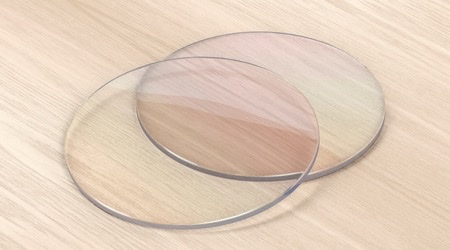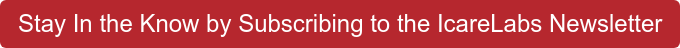# ABO Optical Formulas

Here at IcareLabs, we pride ourselves in making learning a priority. Our sales, marketing, and customer service teams are constantly utilizing different resources to stay up to date with current products. This allows us to share with our customers the benefits of these new products as they roll out.

Recently, we had to opportunity to send a handful of our staff back to school with weekly classes getting them ready to pass the ABO certification test. While I have been in the industry for well over 25 years, I went ahead and jumped at this opportunity and took the class. It is true that you are never too old to learn and I sure was reminded of how much I have forgotten over the years. The old adage of "use it or lose it" was proven to be correct for me.

One of the key reminders for me was some of the optical formulas that we used often back in the day. With modern technology, it is easier just to plug the parameters into a program and let it do the work for us. While this is true, I do believe that knowing these formulas and knowing how to use them will make us better at our jobs. It gives us a better understanding of optical principles that we all should know.

I figured that if I enjoyed being reminded of these formulas, maybe someone else out there would appreciate a cheat sheet with the some basic optical math formulas.

Decentration and Blank Size

This is an important formula for those who are edging stock lenses as this will let you know how large of ablank is needed for a frame. You will need to know a couple of key things before you can start:

1. Find the frame PD by adding the "A" measurement with the "DBL" measurement.
2. Calculate the effective diameter (ED) of the frame by simply finding the longest radius from the geometrical center of the lens and multiply it by two.

To calculate the total decentration (TD), you will want to take the patient's PD and subtract it from the frame PD (FPD).

To find the minimum blank size, all you need to do is add the TD to the ED measurement.

In conclusion, the formula is FPD - PD = TD and then min. blank size is ED + TD
• Example - We have a frame that is a 58/18 with and ED of 62. Patient has a PD is 65.
1. Find the frame PD using: A + DBL = FPD, so 58 + 18 = 76
2. Calculate the total decentration using: FPD - PD = TD, so 76 - 65 = 11
3. Find the minimum blank size using: ED + TD, so 62 + 11 = 73.
• You will need a minimum blank size for 73mm in order for this to cut out.

Prentice's Rule

This formula is used for calculating the amount of prism that is being induced in a pair of lenses or to find out how much to move the OC to induce a specific amount of prism.

In its original form, "Prentice's Rule" was calculated in centimeters. However, nobody uses centimeters in optical anymore so we will use a formula that will convert to millimeters.

First, let's explain what each letter in our formula represents:

• P = Prism
• H = Height or length, amount of distance from the OC
• D = Power of the lens
To figure the amount of prism being induced, the formula would be P = H x D / 10
• For example, let's say the PD was off by 2mm on a lens that was a -5.00
1. Take your formula, P = H x D / 10, and plug in the information that we have.
• In this case, H = 2 and D = 5.00
• So, P = 2 x 5 / 10
• The answer would be 1D of prism. Which means 1D of prism is being induced on a -5.00 lens that is 2mm off on the PD.

To figure out how many millimeters to move a lens to induce a specific amount of prism you would want to flip the formula to H = P / D X 10.

• For example, if you have a -6.00 stock lens and wanted to induce 3 diopters of prism
1. Plug in the information that you already have into your formula. In this case, we know that D = 6.00 and P = 3
2. So, H = 3 / 6 x 10
• So, you would need to move the OC height 5mm in order to induce 3D of prism.

Slab Off

Once you know Prentice's Rule, figuring out the amount of prism to use for a slab off is pretty simple. However, you may want to get your calculator ready.

This is the process of finding the prism at a given point in two lenses of a pair of glasses. This will involve the optical cross and finding the true power of a lens at the desired meridian. Then figuring the prism in each lens and the difference between them.

• For example, the powers of a pair of glasses are OD: -1.00 -4.50 x090 and OS: -3.50 -2.00 x090. The patient is going into a bifocal. The OC difference from the distance to the near is 12mm. Howmuch slab off is needed in this job?

1. Use the Prentice's Rule formula to find the amount of induced prism in each eye. Remember: for this example, we need to find the power at the 90 since the OC is what is being moved.
• OD: P = 12 x 1.00 / 10 = 1.2D of prism
• OD: P = 12 x 3.50 / 10 = 4.2D of prism
2. Subtract the two to find the total slab off.
• 4.2 - 1.2 = 3.0D of slab needed

To determine which eye the slab will need to go on, always remember that it will go on the most minus or least plus eye.

Nominal Power Formula

This is simply figuring the power of the lens based upon the front and back curves.

The formula for this is very simple. It is Front Curve (F) + Back Curve (B) = Power of Lens (P)

• As an example, we know that a lens has a base curve of +6.50 and the back curve is -3.00, what is the power of a lens?
1. Plug in the information that we know into our formula.
• +6.50 + (-3.00) = +3.50

For this example, we know that the power of the lens is +3.50.

As with all algebraic equations, you can adjust this formula to find other information.

• For instance, you are given the power of +2.00 and the front curve of +4.00, what is the back curve of this lens?
1. Adjust your equation so that you are calculating for the back curve of a lens. So, B = P - F.
2. Plug in the information that you are provided with.
• +2.00 - 4.00 = -2.00

We know that the back curve of this lens is -2.00.

Focal Length

This one is interesting and about totally useless. I cannot think of a time when I used this in my career, but it's a cool concept and an optical theory worth knowing. For this formula, focal length will always be in meters.

• To figure focal length (F) from power of lens (D)• F = 1/D
• To figure power from focal length
• D = 1/F

I hope that this can be a reminder to our more experienced readers and a training tool for others. Either way, feel free to print and save it as a resource. You never know when some of these formulas could come in handy.